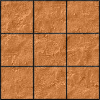#### You may also like### Pyramids

What are the missing numbers in the pyramids?### Paving the Way

A man paved a square courtyard and then decided that it was too small. He took up the tiles, bought 100 more and used them to pave another square courtyard. How many tiles did he use altogether?### Chess

What would be the smallest number of moves needed to move a Knight from a chess set from one corner to the opposite corner of a 99 by 99 square board?

# The Simple Life

##### Age 11 to 14 Challenge Level:

For each of the pairs below, there are whole number multiples that combine to give $5x + 8y$:

$$(x + y) \quad (x + 2y)$$$$(x + y) \quad (x + 4y)$$$$(x + 2y) \quad (2x + 3y)$$$$(2x + 3y) \quad (x + y)$$$$(x – 2y) \quad (x + 4y)$$$$(x + y) \quad (x – 2y)$$$$(x + 2y) \quad (x + 4y)$$